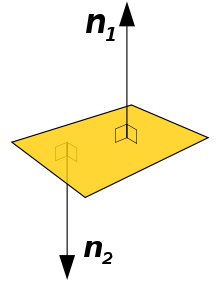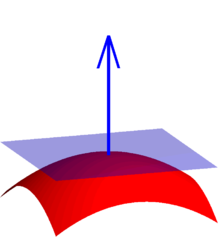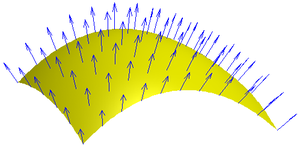Surface normal

﻿
Surface normalA polygon and two of its normal vectorsA normal to a surface at a point is the same as a normal to the tangent plane to that surface at that point.

A surface normal, or simply normal, to a flat surface is a vector that is perpendicular to that surface. A normal to a non-flat surface at a point P on the surface is a vector perpendicular to the tangent plane to that surface at P. The word "normal" is also used as an adjective: a line normal to a plane, the normal component of a force, the normal vector, etc. The concept of normality generalizes to orthogonality.

In the two-dimensional case, a normal line perpendicularly intersects the tangent line to a curve at a given point.

The normal is often used in computer graphics to determine a surface's orientation toward a light source for flat shading, or the orientation of each of the corners (vertices) to mimic a curved surface with Phong shading.

Calculating a surface normal

For a convex polygon (such as a triangle), a surface normal can be calculated as the vector cross product of two (non-parallel) edges of the polygon.

For a plane given by the equation ax + by + cz + d = 0, the vector (a,b,c) is a normal.

For a plane given by the equation$\mathbf{r}(\alpha, \beta) = \mathbf{a} + \alpha \mathbf{b} + \beta \mathbf{c}$,

i.e., a is a point on the plane and b and c are (non-parallel) vectors lying on the plane, the normal to the plane is a vector normal to both b and c which can be found as the cross product$\mathbf{b}\times\mathbf{c}$.

For a hyperplane in n+1 dimensions, given by the equation$\mathbf{r} = \mathbf{a}_0 + \alpha_1 \mathbf{a}_1 + \cdots + \alpha_n \mathbf{a}_n$,

where a0 is a point on the hyperplane and ai for i = 1, ... , n are non-parallel vectors lying on the hyperplane, a normal to the hyperplane is any vector in the null space of A where A is given by$A = [\mathbf{a}_1 \dots \mathbf{a}_n]$.

That is, any vector orthogonal to all in-plane vectors is by definition a surface normal.

If a (possibly non-flat) surface S is parameterized by a system of curvilinear coordinates x(s, t), with s and t real variables, then a normal is given by the cross product of the partial derivatives${\partial \mathbf{x} \over \partial s}\times {\partial \mathbf{x} \over \partial t}.$

If a surface S is given implicitly as the set of points (x,y,z) satisfying F(x,y,z) = 0, then, a normal at a point (x,y,z) on the surface is given by the gradient$\nabla F(x, y, z).$

since the gradient at any point is perpendicular to the level set, and F(x,y,z) = 0 (the surface) is a level set of F.

For a surface S given explicitly as a function f(x,y) of the independent variables x,y (e.g., f(x,y) = a00 + a01y + a10x + a11xy), its normal can be found in at least two equivalent ways. The first one is obtaining its implicit form F(x,y,z) = zf(x,y) = 0, from which the normal follows readily as the gradient$\nabla F(x, y, z)$.

(Notice that the implicit form could be defined alternatively as

F(x,y,z) = f(x,y) − z;

these two forms correspond to the interpretation of the surface being oriented upwards or downwards, respectively, as a consequence of the difference in the sign of the partial derivative$\partial{F}/\partial{z}$.) The second way of obtaining the normal follows directly from the gradient of the explicit form,$\nabla f(x, y)$;$\nabla F(x, y, z) = \hat{\mathbf{k}} - \nabla f(x, y)$, where$\hat{\mathbf{k}}$ is the upward unit vector.

If a surface does not have a tangent plane at a point, it does not have a normal at that point either. For example, a cone does not have a normal at its tip nor does it have a normal along the edge of its base. However, the normal to the cone is defined almost everywhere. In general, it is possible to define a normal almost everywhere for a surface that is Lipschitz continuous.

Hypersurfaces in n-dimensional space

The definition of a normal to a surface in three-dimensional space can be extended to n − 1-dimensional hypersurfaces in a n-dimensional space. A hypersurface may be locally defined implicitly as the set of points$\scriptstyle(x_1, x_2, \ldots, x_n)$ satisfying an equation$F(x_1, x_2, \ldots x_n) = 0$, where F is a given scalar function. If F is continuously differentiable, then the hypersurface obtained is a differentiable manifold, and its hypersurface normal can be obtained from the gradient of F, in the case it is not null, by the following formula$\nabla F(x_1, x_2, \ldots, x_n) = \left( \tfrac{\partial F}{\partial x_1}, \tfrac{\partial F}{\partial x_2}, \ldots, \tfrac{\partial F}{\partial x_n} \right)$

Uniqueness of the normalA vector field of normals to a surface

A normal to a surface does not have a unique direction; the vector pointing in the opposite direction of a surface normal is also a surface normal. For a surface which is the topological boundary of a set in three dimensions, one can distinguish between the inward-pointing normal and outer-pointing normal, which can help define the normal in a unique way. For an oriented surface, the surface normal is usually determined by the right-hand rule. If the normal is constructed as the cross product of tangent vectors (as described in the text above), it is a pseudovector.

Transforming normals

When applying a transform to a surface it is sometimes convenient to derive normals for the resulting surface from the original normals. All points P on tangent plane are transformed to P′. We want to find n′ perpendicular to P. Let t be a vector on the tangent plane and Ml be the upper 3x3 matrix (translation part of transformation does not apply to normal or tangent vectors).$t' = M_l \times t$$n^T \times t = n^T \times M_l^{-1} M_l \times t$$n^T \times t = n^T \times M_l^{-1} M_l \times t = (M_l^{-1T} \times n)^T (M_l \times t)$$n^T \times t = (M_l^{-1T} \times n)^T \times t'$$n' = M_l^{-1T} \times n$

So use the inverse transpose of the linear transformation (the upper 3x3 matrix) when transforming surface normals.

Normal in geometric optics

The normal is an imaginary line perpendicular to the surface of an optical medium. The word normal is used here in the mathematical sense, meaning perpendicular. In reflection of light, the angle of incidence is the angle between the normal and the incident ray. The angle of reflection is the angle between the normal and the reflected ray. That Normal force will then Be perpendicular to the surface

Wikimedia Foundation. 2010.

Look at other dictionaries:

• surface normal — paviršiaus normalė statusas T sritis fizika atitikmenys: angl. normal to a surface; surface normal vok. Flächennormale, f rus. нормаль к поверхности, f pranc. normale à la surface, f …   Fizikos terminų žodynas

• surface normal — noun A unit vector at any given point P of a surface M which is perpendicular to the tangent plane T(M) of M at P. For a surface with parametrization , it is given by :: :where the sign can be chosen at will …   Wiktionary

• Normal mapping — used to re detail simplified meshes. In 3D computer graphics, normal mapping, or Dot3 bump mapping , is a technique used for faking the lighting of bumps and dents. It is used to add details without using more polygons. A common use of this… …   Wikipedia

• Surface second harmonic generation — is a method for probing interfaces in atomic and molecular systems. In second harmonic generation (SHG), the light frequency is doubled, essentially converting two photons of the original beam of energy E into a single photon of energy 2 E as it… …   Wikipedia

• Normal — may refer to: Normality (behavior), conformance to an average Norm (sociology), social norms, expected patterns of behavior studied within the context of sociology Normal distribution (mathematics), the Gaussian continuous probability… …   Wikipedia

• Surface freezing — is the appearance of long range crystalline order in a near surface layer of a liquid. The surface freezing effect is opposite to a far more common surface melting, or premelting. Surface Freezing was experimentally discovered in melts of alkanes …   Wikipedia

• Surface integral — In mathematics, a surface integral is a definite integral taken over a surface (which may be a curved set in space); it can be thought of as the double integral analog of the line integral. Given a surface, one may integrate over it scalar fields …   Wikipedia

• Normal (mathematics) — In mathematics, normal can have several meanings:* Surface normal, a vector (or line) that is perpendicular to a surface. * Normal component, the component of a vector that is perpendicular to a surface. ** Normal curvature, of a curve on a… …   Wikipedia

• normal to a surface — paviršiaus normalė statusas T sritis fizika atitikmenys: angl. normal to a surface; surface normal vok. Flächennormale, f rus. нормаль к поверхности, f pranc. normale à la surface, f …   Fizikos terminų žodynas

• normal — normal, ale, aux [ nɔrmal, o ] adj. et n. f. • 1753; verbe normal h. XVe; lat. normalis, de norma « équerre » 1 ♦ Math. Droite normale, ou n. f. la normale à une courbe, à une surface en un point : droite perpendiculaire à la tangente, au plan… …   Encyclopédie Universelle